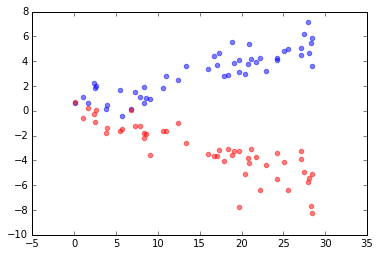Prev: Customizing the plot       |       Next: Multiple plots

Common plots¶

• There are a number of commonly used plots that you should learn how to plot with matplotlib; and we show you how to do it here.
• In the examples we use ad hoc constructed numpy arrays to represent data. When you import data from external files you will replace these data objects with your own data collections.
• Tha plots presented are:
1. Bar chart
2. Pie chart
3. Histogram
4. Box-and-Whisker plot
5. Scatter plot

Bar chart¶

• Bar chart is a simple way for presenting relative frequencies. Bars in the bar chart represent categorical data, and so, a bar chart is used typically to display quantities that fall into different categories. If you want to present the value distribution of a quantitative variable use a histogram instead (see further below).
• To build a bar plot in matplotlib.pyplot use the bar() method

Example¶

• Scenario: You are a biologist measuring turtle species on an isolated island. You find that turtles belong to five different species (denoted as 'SP1',...,'SP5'). Built a bar plot to show the frequency distribution of turtles over the five species. Additionally, present the data that you collected on another island (same species) in the same plot.
In :
import numpy as np
import matplotlib.pyplot as plt
%matplotlib inline

# preparation
N = 5
barloc = np.arange(N)                        # the bar locations
width = 0.25                                 # the width of the bars
plt.xticks(barloc+width, ('SP1', 'SP2', 'SP3', 'SP4', 'SP5'))
plt.yticks(np.arange(0, 100, 10))

# frequencies on island-1
turt = np.array([10, 30, 140, 5, 25])       # measurements of turtle species on island
turtfreq = turt*100/sum(turt)               # frequencies of turtle species on island (bar height)

# frequencies on another island-2
turt2 = np.array([25, 15, 70, 45, 18])      # measurements of turtle species on island-2
turtfreq2 = turt2*100/sum(turt2)                # frequencies of turtle species on island-2 (bar height)

# plotting
b1 = plt.bar(barloc, turtfreq, width, color='c', yerr=2)
b2 = plt.bar(barloc+width, turtfreq2, width, color='r', yerr=1.5)

# legend
plt.ylabel('% Frequencies')
plt.title('Turtle Species Frequency Distribution')
plt.legend((b1, b2), ('Island-1', 'Island-2'))
Out:
<matplotlib.legend.Legend at 0x7961b00>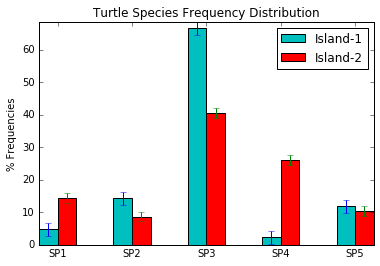Pie chart¶

• A pie chart has the form of a circle divided into circular sectors ("slices") that display the proportion of various parts within the entire entity they constitue.
• The advantage of using a pie chart is its intuitiveness, especially when one or two parts are significanly larger(s) than the others. It should be avoided however when the graph includes many small parts that require detailed display.

Example¶

• Scenario: Similar as above but now a pie chart of frequencies is required
In :
import numpy as np
import matplotlib.pyplot as plt
%matplotlib inline

# plotting
turt = np.array([10, 30, 140, 5, 25])
turtfreq = turt/sum(turt)

p1 = plt.pie(turtfreq, explode=[0,0,0.25,0,0], labels=['SP1','SP2','SP3','SP4','SP5'],
colors=('w', 'g', 'y', 'b', 'm'), autopct='%.2f', shadow=True)

# legend
plt.title('Turtle Species Frequency Distribution')
Out:
<matplotlib.text.Text at 0x7d75a20>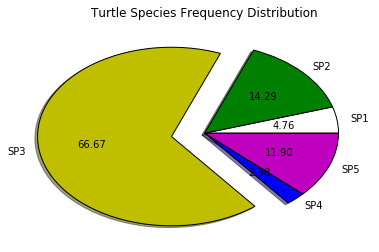Histogram¶

• A histogram is one of the most important and widely used graphical data representation, displaying the measurement distribution of a quantitative variable. Thus, the horizontal axis (x-axis) of a histogram is divided in value intervals ("bins") where measurements are classified while the vertical (y-axis) shows frequencies of measurement or probabilities (if the distribution is normalized).
• A fact emphasizing the importance of displaying information with a histogram is that it is included in the so-called "Seven Basic Tools of Quality"

Example¶

• Scenario: A metereologist making a hundred temprature measurements wants to present them in the form of a histogram.
• The following code shows one possible simple implementation. The pyplot hist() method takes several arguments and can be highly customized and given a complex layout.
In :
import numpy as np
import matplotlib.mlab as mlab
import matplotlib.pyplot as plt
%matplotlib inline

# setting up an ad-hoc distribution for temprature values
n = 100                                            # number of measurements
mn = 21.5                                          # the mean of the temperature distribution
std = 2.5                                          # the standard deviation of the temperature distribution
temp = std * np.random.randn(n) + mn               # 'temp' is a numpy array with n values

n, bins, patches = plt.hist(temp, color='cyan', alpha=0.5)
# n: the value in each bin
# bins: the intervals in which the histogram is divided; default = 10
# alpha: opacity of the plot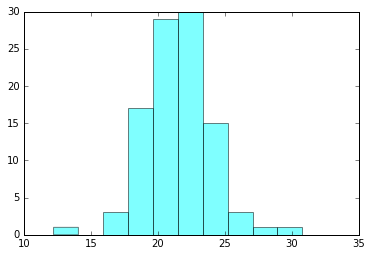• A more elaborate version of hist()
In :
import numpy as np
import matplotlib.mlab as mlab
import matplotlib.pyplot as plt
%matplotlib inline

# setting up an ad-hoc distribution for temprature values
# normally the 'temp' array would be filled in with data read from external file
n = 100                                            # number of measurements
mn = 21.5                                          # the mean of the temperature distribution
std = 2.5                                          # the standard deviation of the temperature distribution
temp = std * np.random.randn(n) + mn               # 'temp' is now a numpy array with n values
tbins = 20

n, bins, patches = plt.hist(temp, tbins, normed=True, color='green', alpha=0.5)
# tbins: we set a specific number of bins
# normed=True: now bin heights are normalized (area = integral of the histogram equals 1).

# Labels
plt.xlabel('Temperature')
plt.ylabel('Probability')
plt.title('Temperature measurement')

# Normal distro
y = mlab.normpdf(bins, mn, std)
plt.ylim(0,0.20)
plt.plot(bins, y, 'r--')
Out:
[<matplotlib.lines.Line2D at 0x7e827f0>]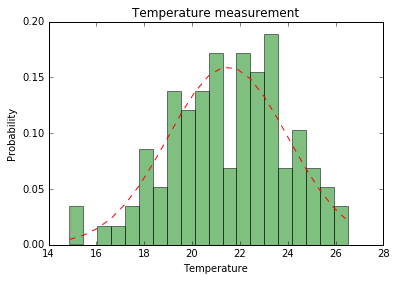Box-and-Whisker plot¶

• Box-and-whisker plot offers a compact form of representing a distribution based on its quartiles. If 'σ' is the distribution standard deviation, then the "box" upper and lower sides indicate the 3rd and 1st quartile respectively (see the plot in the example below). Additionally, the band in the box indicates the median of the distribution, while the "whiskers" (upper and lower) usually (but can be changed) indicate the -3σ and +3σ points of the distribution.

Example¶

• Scenario: The same temprature distribution as in the above histogram scenario but now presented in a box-and-whisker plot
In :
import numpy as np
import matplotlib.pyplot as plt
%matplotlib inline

# np.random.seed(937)
# data = np.random.lognormal(size=(37, 4), mean=1.5, sigma=1.75)
# labels = list('ABCD')
# fs = 10  # fontsize

n = 100                                            # number of measurements
mn = 21.5                                          # the mean of the temperature distribution
std = 2.5                                          # the standard deviation of the temperature distribution
temp = std * np.random.randn(n) + mn               # 'temp' is now a numpy array with n values

n = 100
mn = 24.5
std = 2.0
temp2 = std * np.random.randn(n) + mn

#temps = np.array([temp, temp2])
list2 = list(temp2)
list1 = list(temp)

temps = [list1,list2]

di = plt.boxplot(temps)
plt.ylim(15,30)
Out:
(15, 30)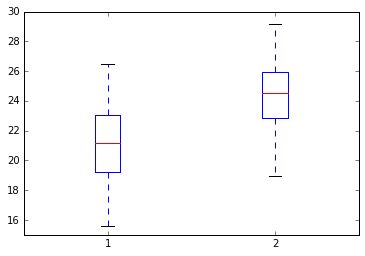Scatter plot¶

• Scatter plot is a bivariate distribution plot, displaying how the output variable changes in response to input variable changes.

Example¶

• Scenario: The data below have been set up so that x and y1 variables have positive correlation (b1>0) while x and y2 have negative (b2<0)
In :
import numpy as np
import matplotlib.pyplot as plt
%matplotlib inline

N=50
b1 = 0.2
b2 = -0.2
x = 30*np.random.sample(N)
y1 = b1*x+np.random.randn(N)
y2 = b2*x+np.random.randn(N)

plt.scatter(x, y1, color='blue', alpha=0.5)

plt.scatter(x, y2, color='red', alpha=0.5)
Out:
<matplotlib.collections.PathCollection at 0x7fb6f60>